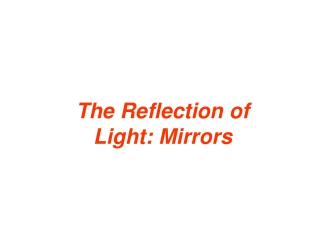DownloadDownload PresentationThe Reflection of Light: Mirrors

# The Reflection of Light: Mirrors

Download Presentation## The Reflection of Light: Mirrors

- - - - - - - - - - - - - - - - - - - - - - - - - - - E N D - - - - - - - - - - - - - - - - - - - - - - - - - - -
##### Presentation Transcript

1. The Reflection of Light: Mirrors

2. 25.2 The Reflection of Light LAW OF REFLECTION The incident ray, the reflected ray, and the normal to the surface all lie in the same plane, and the angle of incidence equals the angle of reflection.

3. 25.2 The Reflection of Light In specular reflection, the reflected rays are parallel to each other.

4. 25.3 The Formation of Images by a Plane Mirror • The person’s right hand becomes • the image’s left hand. • The image has three properties: • It is upright. • It is the same size as you are. • The image is as far behind the m • mirror are you are in front of it.

5. 25.3 The Formation of Images by a Plane Mirror The geometry used to show that the image distance is equal to the object distance.

6. 25.3 The Formation of Images by a Plane Mirror Conceptual Example 1 Full-Length Versus Half-Length Mirrors What is the minimum mirror height necessary for her to see her full image?

7. 25.4 Spherical Mirrors If the inside surface of the spherical mirror is polished, it is a concave mirror. If the outside surface is polished, is it a convex mirror. The law of reflection applies, just as it does for a plane mirror. The principal axis of the mirror is a straight line drawn through the center and the midpoint of the mirror.

8. 25.4 Spherical Mirrors A point on the tree lies on the principal axis of the concave mirror. Rays from that point that are near the principal axis cross the axis at the image point.

9. 25.4 Spherical Mirrors Light rays near and parallel to the principal axis are reflected from the concave mirror and converge at the focal point. The focal length is the distance between the focal point and the mirror.

10. 25.4 Spherical Mirrors The focal point of a concave mirror is halfway between the center of curvature of the mirror C and the mirror at B.

11. 25.4 Spherical Mirrors When paraxial light rays that are parallel to the principal axis strike a convex mirror, the rays appear to originate from the focal point.

12. 25.5 The Formation of Images by Spherical Mirrors CONCAVE MIRRORS This ray is initially parallel to the principal axis and passes through the focal point. This ray initially passes through the focal point, then emerges parallel to the principal axis. This ray travels along a line that passes through the center.

13. 25.5 The Formation of Images by Spherical Mirrors When an object is located between the focal point and a concave mirror, and enlarged, upright, and virtual image is produced.

14. 25.5 The Formation of Images by Spherical Mirrors CONVEX MIRRORS Ray 1 is initially parallel to the principal axis and appears to originate from the focal point. Ray 2 heads towards the focal point, emerging parallel to the principal axis. Ray 3 travels toward the center of curvature and reflects back on itself.

15. 25.5 The Formation of Images by Spherical Mirrors The virtual image is diminished in size and upright.

16. 25.6 The Mirror Equation and Magnification

17. 25.6 The Mirror Equation and Magnification These diagrams are used to derive the mirror equation.

18. 25.6 The Mirror Equation and Magnification Summary of Sign Conventions for Spherical Mirrors